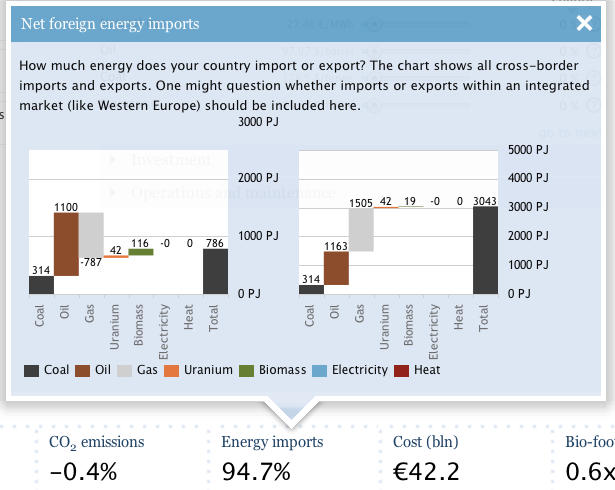# Import calculationsThe dashboard shows the percentage of primary energy consumption that is imported and the pop-up shows a breakdown of the net energy imports per carrier group. International marine and aviation bunkers are not included. This page describes the calculation method.

## How import is calculated#

The ETM is demand driven, which means it calculates how much primary energy is required to meet the final consumption. For each area, the input data includes domestic production curves (see below) that describe the amount of energy that is produced/extracted domestically for all years up to 2050. Depending on the scenario year, the amount of domestically produced/extracted energy may therefore differ.

When the domestic demand for a carrier exceeds domestic production/extraction the remainder is imported. Vice versa, when domestic production/extraction of a carrier is higher than what is required, the excess amount is exported.

## Carriers#

This is determined for the following primary energy carriers:

• Coal
• Oil
• Crude oil
• Diesel
• Gasoline
• Kerosine
• LPG
• Gas
• Natural gas
• LNG
• Uranium
• Electricity
• Biomass
• Bio-diesel
• Bio-ethanol
• Bio-oil
• Biofuel (Deprecated)
• Greengas
• Waste (All waste including non-biogenic)
• Algue diesel
• Wood pellet
• Biocoal
• Heat

(Lignite cannot be imported from a neighboring country, as this would not be energy efficient. All lignite is therefore assumed to be locally available. For each country it is defined whether or not lignite is available for production of electricity.)

All converters that import energy are grouped together in a group named `energy_import` and all converters that export energy are in the group `energy_export` The total net import is the sum of all converters in the `energy_import` group minus those in the `energy_export` group. To calculate how large the total imported energy is compared to the total energy use of the area, the total amount of energy import is divided by the total primary energy consumption of the region. The total primary energy consumption includes the conversion losses incurred for the generation of electricity that is exported.

## Domestic production curves#

For energy carriers that can be produced locally, production curves are used as input to the model calculations. The available amount of oil in an area for example, depends on the value that is supplied in this curve for the scenario year.